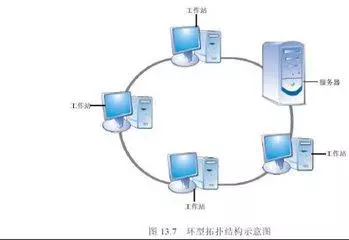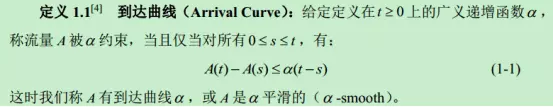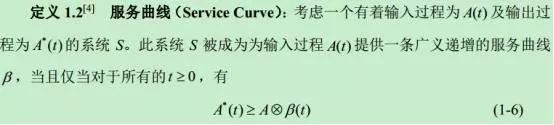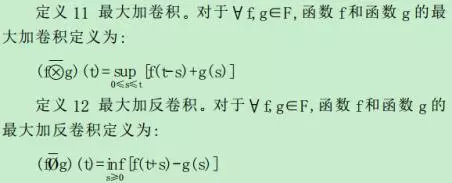• 随机网络演算，liu Yong和Jiang Yuming写的。随机网络演算定义了统计边界，允许实际情况以一定的概率超过统计边界，从而得到了系统的随机服务质量保证，避免了确定性网络演算过于保守带来的资源浪费问题。数学
• 随机网络演算，网络性能分析工具 网络基本性能分析、流量控制、节点的调度、缓存、延迟的定量估计 确定性网络演算理论：P{服务质量不比预定目标差}=1 随机性网络演算理论：P{服务质量比预定目标差}≤ε
•研究论文
•研究论文
• 针对控制网络的特殊性,总结、拓展了网络演算理论,结合控制网络拓扑结构以及数据调度策略,得出了基于网络演算理论的NCS网络一般性延迟模型及延迟上界, 使得对NCS确定性延迟的研究更符合完备性的要求.</p>
•研究论文
• 深入研究了网络演算在网络资源预留中的应用方法，推导出了基于端到端时延约束的有效带宽和基于缓冲区长度约束的等效容量计算公式，分析了给定到达曲线和服务曲线条件下网络性能的计算方法。通过具体的应用实例，验证...服务质量 网络性能分析 资源预留
• 网络演算(Networkcalculus)是一种基于非线性代数的确定性排队理论，目前已广泛应用于计算机网络建模与性能分析，特别是为计算延迟和积压等端到端性能参数的确界提供了有效工具。 网络演算的研究工作可分为理论研究与...
中文释义
网络演算(Networkcalculus)是一种基于非线性代数的确定性排队理论，目前已广泛应用于计算机网络建模与性能分析，特别是为计算延迟和积压等端到端性能参数的确界提供了有效工具。
网络演算的研究工作可分为理论研究与应用研究。理论研究的研究对象是网络演算的数学模型，是网络演算得以广泛应用的基础。理论研究的每一步进展都可以带动大批实际应用。由于网络演算属于跨学科的研究领域，涉及较多数学理论，因此理论研究的进展相对于应用而言较为缓慢。
网络演算作为一种网络性能分析工具，按照普遍的观点可以分为确定性网络演算和随机网络演算。确定性网络演算比较简单，目的是得到网络性能的最坏边界。随机网络演算的目的是为网络提供随机服务质量保障，要考虑网络数据流的随机突发，自相识等特性，以及网络信道的接入拥塞物理信道的衰落等因素，因此相对应用起来比较复杂，因此许多研究人员也运用了不同的数学方法和不同的数学表达方式来扩充随机网络演算。
网络演算的主要工具是到达曲线和服务曲线。
网络演算主要是用来得到以下5个结果：
1）数据流叠加
2）输入输出的关系
3）网络延时和积压性质
4）节点串联
5）剩余服务曲线
https://baike.baidu.com/item/%E7%BD%91%E7%BB%9C%E6%BC%94%E7%AE%97
http://gb.oversea.cnki.net/KCMS/detail/detail.aspx?filename=2010147286.nh&dbcode=CDFD&dbname=CDFDREF
英文释义
Network calculus is “a set of mathematical results which give insights into man-made systems such as concurrent programs, digital circuits and communication networks.” Network calculus gives a theoretical framework for analysing performance guarantees in computer networks. As traffic flows through a network it is subject to constraints imposed by the system components, for example:

traffic shapers (leaky buckets)
congestion control
background traffic

These constraints can be expressed and analysed with network calculus methods. Constraint curves can be combined using convolution under min-plus algebra. Network calculus can also be used to express traffic arrival and departure functions as well as service curves.
The calculus uses “alternate algebras … to transform complex non-linear network systems into analytically tractable linear systems.”
Currently, there exists two branches in network calculus: one handling deterministic bounded, and one handling stochastic bounds .
System modelling
Modelling flow and server
In network calculus, a flow is modelled as cumulative functions A, where A(t) represents the amount of data (number of bits for example) send by the flow in the interval [0,t). Such functions are non-negative and non-decreasing. The time domain is often the set of non negative reals.
Arrival and departure curve at ingress and egress (入口和出口) of a server.
$A:\mathbb {R} ^{+}\rightarrow \mathbb {R} ^{+}}A:{\mathbb R}^{+}\rightarrow {\mathbb R}^{+$
$\forall u,t\in \mathbb {R} ^{+}:u
A server can be a link, a scheduler, a traffic shaper, or a whole network. It is simply modelled as a relation between some arrival cumulative curve A and some departure cumulative curve D. It is required that A ≥ D, to model the fact that the departure of some data can not occur before its arrival.
Modelling backlog and delay
Given some arrival and departure curve A and D, the backlog at any instant t, denoted b(A,D,t) can be defined as the difference between A and D. The delay at t, d(A,D,t) is defined as the minimal amount of time such that the departure function reached the arrival function. When considering the whole flows, the supremum of these values is used.Horizontal and vertical deviation between arrival and departure cumulative curves
$b(A,D,t):=A(t)-D(t)}b(A,D,t):=A(t)-D(t$
$d(A,D,t):=\inf \left\{d\in \mathbb {R} ^{+}~s.t.~D(t+d)\geq A(t)\right\}}d(A,D,t):=\inf \left\{d\in {\mathbb R}^{+}~s.t.~D(t+d)\geq A(t)\right\$
$b(A,D):=\sup _{t\geq 0}\left\{A(t)-D(t)\right\}}b(A,D):=\sup _{{t\geq 0}}\left\{A(t)-D(t)\right\$
$d(A,D):=\sup _{t\geq 0}\left\{\inf \left\{d\in \mathbb {R} ^{+}~s.t.~D(t+d)\geq A(t)\right\}\right\}}d(A,D):=\sup _{{t\geq 0}}\left\{\inf \left\{d\in {\mathbb R}^{+}~s.t.~D(t+d)\geq A(t)\right\}\right\$
In general, the flows are not exactly known, and only some constraints on flows and servers are known (like the maximal number of packet sent on some period, the maximal size of packets, the minimal link bandwidth). The aim of network calculus is to compute upper bounds on delay and backlog, based on these constraints. To do so, network calculus uses the min-plus algebra.
Min-plus algebra
In filter theory and linear systems theory the convolution of two functions $f$ and $g$ is defined as
$(f\ast g)(t):=\int _{0}^{t}f(\tau )\cdot g(t-\tau )d\tau$
In min-plus algebra the sum is replaced by the minimum respectively infimum operator and the product is replaced by the sum. So the min-plus convolution of two functions $f$ and $g$ becomes
$(f\otimes g)(t):=\inf _{0\leq \tau \leq t}\left\{f(\tau )+g(t-\tau )\right\}$
e.g. see the definition of service curves. Convolution and min-plus convolution share many algebraic properties. In particular both are commutative and associative.
A so-called min-plus de-convolution operation is defined as
$(f\oslash g)(t):=\sup _{\tau \geq 0}\left\{f(t+\tau )-g(\tau )\right\}$
e.g. as used in the definition of traffic envelopes.
The vertical and horizontal deviations can be expressed in terms of min-plus operators.
$b(f,g)=(f\oslash g)(0)$
$d(f,g)=\inf\{w:(f\oslash g)(-w)\leq 0\}$
Traffic envelopes
Cumulative curves are real behaviours, unknown at design time. What is known is some constraint. Network calculus uses the notion of traffic envelope, also known as arrival curves.
A cumulative function A is said to conform to an envelope (or arrival curve) E, if for all t it holds that
$E(t)\geq \sup _{\tau \geq 0}\{A(t+\tau )-A(\tau )\}=(A\oslash A)(t)$.
Two equivalent definitions can be given
$\forall \tau ,t\in \mathbb {R} ^{+}:A(\tau +t)-A(\tau )\leq E(t)$  --------- (1)
$A\leq A\otimes E$  --------- (2)
Thus, E places an upper constraint on flow A. Such function E can be seen as an envelope that specifies an upper bound on the number of bits of flow seen in any interval of length t starting at an arbitrary τ, cf. eq. (1).
Service curves
In order to provide performance guarantees to traffic flows it is necessary to specify some minimal performance of the server (depending on reservations in the network, or scheduling policy, etc.). Service curves provide a means of expressing resource availability. Several kinds of service curves exists, like weakly strict, variable capacity node, etc. See   for an overview.
Minimal service
Let A be an arrival flow, arriving at the ingress of a server, and D be the flow departing at the egress. The system is said to provide a simple minimal service curve S to the pair (A,B), if for all t it holds that $D(t)\geq (A\otimes S)(t)$.
Strict minimal service
Let A be an arrival flow, arriving at the ingress of a server, and D be the flow departing at the egress. A backlog period is an interval I such that, on any $t ∈ I, A(t)>D(t)$.
The system is said to provide a strict minimal service curve S to the pair (A,B) iff, $\forall s,t\in \mathbb {R} ^{+}$, such that $s\leq t$, if $(s,t]$ is a backlog period, then$D(t)-D(s)\geq S(t-s)$.
If a server offers a strict minimal service of curve S, it also offers a simple minimal service of curve S.
Basic results: Performance bounds and envelope propagation
From traffic envelope and service curves, some bounds on the delay and backlog, and an envelope on the departure flow can be computed.
Let A be an arrival flow, arriving at the ingress of a server, and D be the flow departing at the egress. If the flow as a traffic envelope E, and the server provides a minimal service of curve S, then the backlog and delay can be bounded:
$b(A,D)\leq b(E,S)$
$d(A,D)\leq d(E,S)$
Moreover, the departure curve has envelope $E'=E\oslash S.$
Moreover, these bounds are tight i.e. given some E, and S, one may build an arrival and departure such that $b(A,D) = b(E,S)$ and $v(A,D)=v(E,S)$.
Concatenation / PBOO
Consider a sequence of two servers, when the output of the first one is the input of the second one. This sequence can be seen as a new server, built as the concatenation of the two other ones.
Then, if the first (resp. second) server offers a simple minimal service $S_{1}$ (resp. $S_{2}$), then, the concatenation of both offers a simple minimal service $S_{{e2e}}=S_{1}\otimes S_{2}$.
Sequence of two servers
The proof does iterative application of the definition of service curves $X\geq A\otimes S_{1}$, $D\geq X\otimes S_{2}$ and some properties of convolution, isotonicity ($D\geq (X\otimes S_{2})\otimes S_{1}$), and associativity ($D\geq X\otimes (S_{2}\otimes S_{1}$)).
The interest of this result is that the end-to-end delay bound is not greater than the sum of local delays: $d(E,S_{2}\otimes S_{1})\leq d(E,S_{1})+d(E\oslash S_{1},S_{2}$).
This result is known as Pay burst only once (PBOO).
Tool
There are several tools based on network calculus.

The DiscoDNC is an academic Java implementation of the network calculus framework.
The RTC Toolbox is an academic Java/MATLAB implementation of the Real-Time calculus framework, a theory quasi equivalent to network calculus.
The CyNC tool is an academic MATLAB/Symulink toolbox, based on top of the RTC Toolbox. The tool was developed in 2004-2008 and it is currently used for teaching at Aalborg university.
The RTaW-PEGASE is an industrial tool devoted to timing analysis tool of switched Ethernet network (AFDX, industrial and automotive Ethernet), based on network calculus.
The Network calculus interpreter is an on-line (min,+) interpreter.
The WOPANets is an academic tool combining network calculus based analysis and optimization analysis.
The DelayLyzer is an industrial tool designed to compute bounds for Profinet networks.
DEBORAH is an academic tool devoted to FIFO networks.
NetCalBounds is an academic tool devoted to blind & FIFO tandem networks.
NCBounds is a network calculus tool in Python, published under BSD 3-Clause License. It considers rate-latency servers and token-bucket arrival curves. It handles any topology, including cyclic ones.
The Siemens Network Planner (SINETPLAN) uses network calculus (among other methods) to help the design of a PROFINET network.

Reference
https://en.wikipedia.org/wiki/Network_calculus


展开全文•研究论文
• 为分析融合网络网关节点统计时延性能，根据最小加代数理论中的统计网络演算的相关知识，提出了一种基于改进的GPS调度系统模型，然后利用网络演算理论求解出网关节点统计时延上界。数值结果分析表明，改进的调度模型...
• 摘要：网络演算(Networkcalculus)是一种基于非线性代数的确定性排队理论,目前已广泛应用于计算机网络建模与性能分析,特别是为计算延迟和积压等端到端性能参数的确界提供了有效工具.网络演算的研究工作在近年得到了...
摘要：网络演算(Networkcalculus)是一种基于非线性代数的确定性排队理论,目前已广泛应用于计算机网络建模与性能分析,特别是为计算延迟和积压等端到端性能参数的确界提供了有效工具.网络演算的研究工作在近年得到了迅速发展,现已成为计算机网络的热点研究领域. 网络演算的研究工作可分为理论研究与应用研究.理论研究的研究对象是网络演算的数学模型,是网络演算得以广泛应用的基础.理论研究的每一步进展都可以带动大批实际应用.由于网络演算属于跨学科的研究领域,涉及较多数学理论,因此理论研究的进展相对于应用而言较为缓慢.网络演算中的基本概念如到达曲线和服务曲线的理论背景尚未得到充分研究,网络演算的理论自身也存在不严密之处.采用数学理论分析网络演算,并将网络演算纳入一个严格的理论体系,是一项值得深入研究的工作. 针对网络演算理论研究的不足,论文在现有研究成果的基础上,采用幂等数学理论和离散事件动态系统DEDS(Discrete Event Dynamic System)理论对网络演算进行了研究. 论文提出了基于极大代数的网络演算和二阶时变网络演算.基于极大代数的网络演算在处理变长分组序列以及计算与时间相关的性能参数时具有很大优势,有效的弥补了基于极小代数的网络演算的不足.二阶时变网络演算对网络元素输出流累积函数的差分特征进行了描述,是一种比一阶时变网络演算更强的性能分析工具.采用二阶时变网络演算进行性能分析可以得到更加准确的结果. 论文提出了基于余理论的网络演算数学模型.采用余理论经过简单的推导就可以得出网络演算的主要结论,极大的降低了网络演算理论研究的难度.论文采用余理论解决了网络演算中一些难以解决的问题,这些问题包括贪心整形器的最大输入,窗口流量控制系统的最优化窗口大小.利用余理论能很好的分析如勒让德变换和分组器函数等许多余映射或对偶余映射,进一步扩大了网络演算对计算机网络性能分析的范围和能力. 论文提出了一种抽象网络演算理论.抽象网络演算总结了不同网络演算的共同点,将各种网络演算理论都纳入到了一个全新的理论体系之中,从而为网络演算打下了坚实的理论基础.抽象网络演算没有对双子中的元素赋予任何特殊意义,推导过程也只用到了双子的基本性质,因此简单易懂,并为不同的网络演算提供了通用的分析方法.研究者不需要去研究所有的网络演算就可以掌握网络演算的核心内容.作为抽象网络演算的具体应用,论文提出了区间网络演算,区间网络演算建立在广义增函数双子的区间扩展之上.通过区间演算可以计算网络性能参数的区间界,因而可以处理网络中的不确定性.区间网络演算的提出为网络性能分析提供了一种新的工具,区间演算的复杂程度介于确定性网络演算与随机网络演算之间. 论文采用幂等矩阵理论研究了到达曲线和服务曲线,并定义了到达矩阵和服务矩阵的概念.在这两个概念的基础上提出了矩阵网络演算.论文还根据数据流的生成矩阵的阶数将网络演算分为4类.矩阵网络演算有如下优点:首先,矩阵形式易于理解,在矩阵演算中,极小卷积变成了较为常见的矩阵乘法;其次,可以采用幂等矩阵理论中的成熟结论研究网络演算;最后,矩阵网络演算的提出加深了网络演算与DEDs理论的联系,从而可以用DEDs理论更深入的分析网络演算.展开
展开全文•研究论文
• 为保证周期性实时数据在交换式工业以太网中的服务... 基于网络演算理论推导了交换机对不同类型数据的服务曲 线; 针对一个树型拓扑的交换式工业以太网模型, 利用所推导出的结论计算了实时数据的最大网络时延.</p>
• 网络演算 全文 英文 原版 I AFirstCourseinNetworkCalculus 1 NetworkCalculus 2 ApplicationtotheInternet II MathematicalBackground 3 BasicMin-plusandMax-plusCalculus 4 Min-plusandMax-PlusSystemTheory ...
• 为了保证认知无线电传感器网络（CRSN）的服务质量（QoS），需精确求解所采用协议的性能边界，为此提出了一种基于随机网络演算（SNC）的QoS性能边界分析方法。以CRSN中的和式增加积式减少（AIMD）拥塞控制机制为评估...服务质量
• 确定性网络演算（DNC）是一种网络分析工具，用于提供确定性延迟或带宽限制。 该方案在Mininet中实现了基于DNC的端到端QoS路由算法，其中Open vSwitch为转发交换机，Ryu为远程控制器。 输出端口运行“分层令牌桶”...研究论文
• 网络演算在AFDX网络时延计算中的应用，季晓强，李健，新一代航空数据总线--AFDX（Avionics Full Duplex Switched Ethernet）是基于以太网的实时应用协议，也称作ARINC664，主要用于互连航空飞行器中的��
•研究论文
• 【导读】：服务质量（QOS)一直是各种网络系统中人们最关注的方面之一，而随机网络演算理论是近来发展起来的一种新的网络QOS理论,其可将复杂的网络系统转换成易于分析的模型，提供一定概率服务质量保证，相比确定型...
【导读】：服务质量（QOS)一直是各种网络系统中人们最关注的方面之一，而随机网络演算理论是近来发展起来的一种新的网络QOS理论,其可将复杂的网络系统转换成易于分析的模型，提供一定概率服务质量保证，相比确定型网络演算可以很大程度的提高网络资源利用率，作为一种网络性能分析工具，随机网络演算理论越来越受到众多研究者的关注，并且在近些年来得到了极大的发展和完善。
SNR—随机网络演算理论
【提问】什么是SNR?
SNR中采用的到达曲线和服务曲线是什么？及数学工具有哪些？
SNR研究现今面临哪些挑战？
【正文】：
随机网络演算
1.SNR概述
随着计算机网络技术的飞速发展和网络保证服务的提出,对网络QoS（服务质量)问题的研究成为人们当下研究的热点。在Internet等高速网络领域,经常需要定性或定量分析当前网络负载或设计发生变化时,对整个网络所产生的影响及效果,或者通过对现有网络情况进行推广来预测预期的未来情形。
网络演算是近年来人们对网络通信流问题进行深入研究所取得的一些成果的有机结合,是最小加代数和最大加代数在网络定性和定量分析中的应用所取得的一系列有用结论的集合。网络演算是一种用来处理计算机网络中排队系统的理论，最初由Cruz提出。它主要用于分析网络的端到端时延以及网络中的积压等性能指标，基于这些分析结果，可以通过调整网络系统提供的服务速率、缓存大小等资源来获得期望的服务质量保障。网络演算的核心思想即为将网络中的到达流和网络提供的服务用到达曲线和服务去建模，同时引入新的数学分析工具将复杂的网络系统转换易于分析的模型，对网络的性能参数进行一系列分析，为计算机网络提供一定的服务质量的保证。
网络演算有两个分支：
A:确定型网络演算能够提供确定的服务质量保证，网络资源利用率低。
B:随机网络演算提供一定概率服务质量保证，网络资源利用率相对高，存在难题如丢包分析以及对于多服务系统的分析等。
2.到达曲线和服务曲线
假定我们想要给数据流提供一些保证，这便需要一些具体的网络来支持。作为支持措施的一部分，需要限制源发送流量的速率。这即是引入到达曲线的概念。即约束流量的到达曲线。常见到达曲线类型如下：
1)仿射到达曲线
2)阶梯函数到达曲线
为了提供资源预留，网络节点必须对流量提供一些保障，这一功能由包调度器（packet scheduler）来实现的。具体的包调度方法，由服务曲线（Service Curve） 这个概念来实现。3.SNR中采用的数学工具
最小加代数
最小加代数最初是用于数字电路、通信网络以及制造工业等离散事件系统( Discrete Event Systems)建模的一种数学工具。在最小加代数中，下面两个运算符经常用到：最大加代数4.SNR研究现今面临哪些挑战？
在随机网络演算理论的不断探索与完善的过程中，我们目前仍存在着一些挑战性的问题尚未解决。
难点一：理论运用条件
随机网络演算理论包括了不同的流量模型和服务模型以及在此基础上推导出的一些基本性质。然而，如果对流量模型和服务模型没有附加条件限制，一些关于随机网络演算的结论的应用范围极其有限。例如弱服务曲线由于不具有串联特性就难以用在数据流经过多节点的场景。因此为了更容易的得到期望的分析结果，对于随机到达曲线和随机服务曲线需要更强的定义。那么问题来了：这些附加限制是什么？这些限制对于分析和结果有着什么样的关系？回答好这些问题能够对在什么时候使用什么样的模型从而得到期望的性能分析提供指导。
难点二：网络场景的多样性
确定型网络演算主要应用于流量受限的确定型网络中。对于链路本质上是随机的网络来说，随机网络演算更加适合。无线网络和P2P网络就是两种具有代表性的网络，然而在利用随机网络演算对这类网络进行分析时，将会不可避免的受到这两种网络场景的各种约束和限制。
难点三：丢包分析
丢包是网络服务质量里非常重要的一项，然而目前关于随机服务质量保证的研究主要集中在时延、吞吐量和积压这几个方面。当前大多数关于丢包的研究都是直接近似的用积压分析得到的结果来表示丢包。然而，这种近似通常得到的是非常松的边界。因此，在网络演算的理论框架下直接对丢包行为进行研究并获得相应的丢包边界是非常有必要的一项研究。在确定型的网络演算中，已经有了一些相关的研究。但在随机网络演算中，相关的研究几乎还是空白。
挑战即是机遇，也必将是今后随机网络演算研究的新的发展方向，如何解决这些难题进而不断完善SNR理论，是提高通信网络服务质量的前提。


展开全文• 蜂窝网络中设备间通信访问控制的建模与分析：一种基于网络演算的方法研究论文
• 基于随机网络演算的NB-IoT访问延迟分析与优化研究论文
• 首次将动态交通流量分配拟化为网络负载均衡问题, 使用漏桶理论和网络演算方法, 将交通的流量分配与路径时延转换为一系列极值运算, 结合贪婪算法, 以均衡网络延时为优化目标, 得到交通配流。仿真结果表明, 本模型在...
• 基于网络演算的软件定义车联网服务质量路由算法.pdf
• 使用网络演算对M2M通信的准入控制进行建模和分析研究论文
• 1. Arrival curves 2. Service curves, backlog, delay bounds 3. Playback delay for pre-recorded video
• 运用有利于量化计算的网络演算理论,结合IPv6的特性和流标签、优先级域等优势,提出了一种改进的QoS模型―――RSM 模型,并通过与集成服务和区分服务模型进行对比分析和实例计算,证实了RSM 模型在大规模IP网络中也具有...
• 大数据分析和网络演算使智慧城市中的自动驾驶汽车实现智能管理研究论文
• 使用雾计算和网络演算的软件定义的能源互联网智能计量动态QoS设置研究论文
• 随机网络演算分析全局冗余TSV性能，杜高明，屠庆东，硅通孔（Through Silicon Via，TSV）技术是三维芯片中互联上下层不同模块的主要方法之一，然而由于制造工艺水平的限制，在芯片制作完成��...